# How to Complete a Regression Analysis in Minitab 18

## Why We Use Regression Analysis

When you look at a scatter plot graph like the one above created by plotting the dots from two different axes, you will find that the variables are inversely related, directly related, or show no relation at all.

• Directly Related: If the line you draw to estimate regression seems to be moving from the left to the upper right corner of the scatter plot, the data is said to be directly related.
• Inversely Related: The data is inversely related if it's moving from the left to the lower right corner.
• No Relationship: When the data plots are spread out evenly with no apparent direction, there is no relationship.

### Follow Along With This Tutorial

It‘s nice to have a quick view of the scatter plot, but a relationship between variables can be more accurately determined through regression analysis. In this tutorial, a scattered plot with a regression line will be created. To follow along with this lesson, download this Minitab file. Also, if you don't have the latest version of Minitab, you can download a free trial for the new version here.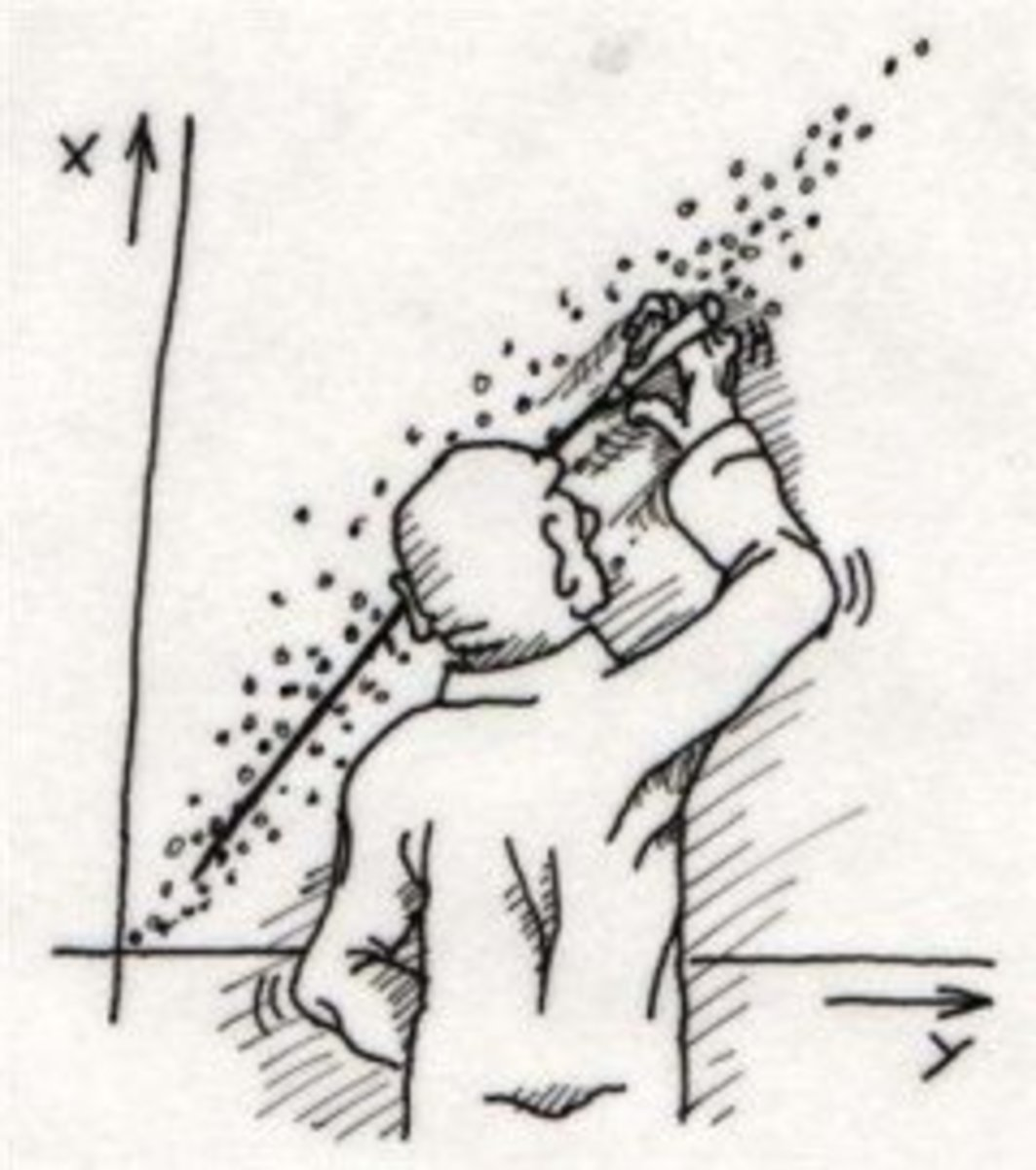Completing regression by hand can be quite tedious. Luckily, we have software like Minitab and Microsoft Excel to calculate problems associated with regression.

## Add Data to Minitab

To add data to Minitab, the data must be entered or pasted into the program from a spreadsheet. The data should come in the form of X and Y separately to be able to complete an analysis.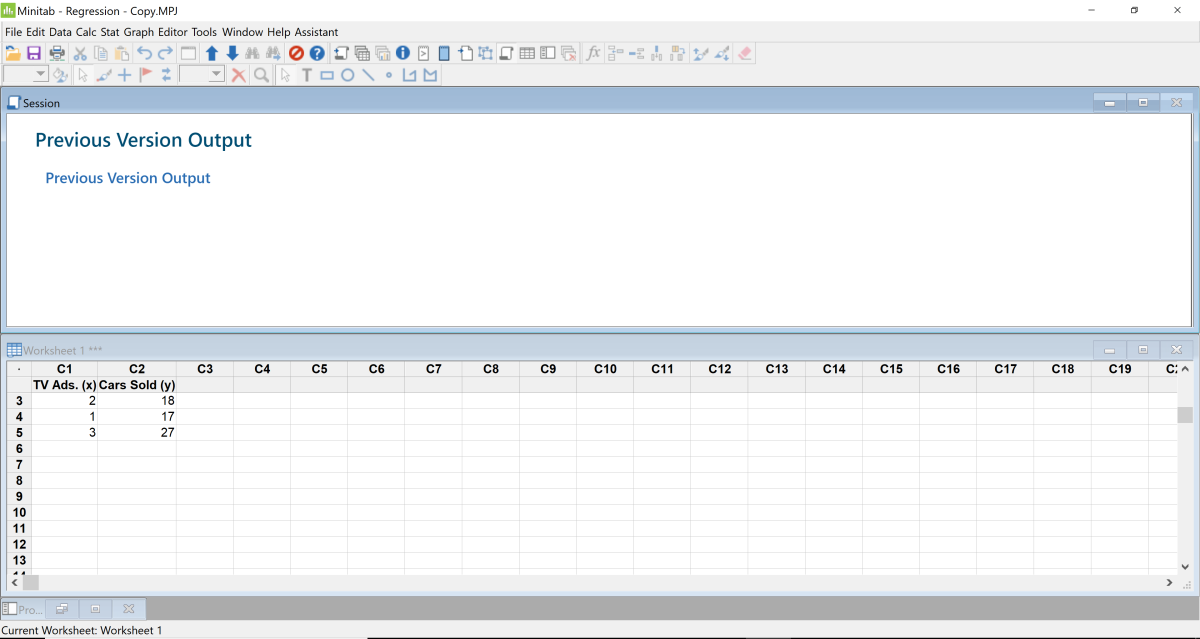The only variable data used for the scattered plot graph or regression analysis are the dependent and independent variables X and Y. Created by Joshua Crowder

## Set up a Scatter Plot With a Regression

The first graph that we need to bring up for regression analysis is a scattered plot graph.

1. To set up this graph, click Graph then Scatterplot.
2. When the scatterplot window appears, select the box labeled "With Regression" and click OK.
3. When the scattered plot with regression window appears, put the cursor in the first row of the Y-axis box, then double click on the Y variable to the left.
4. Next, double click on the X variable and it will populate the X section.
5. A default name will appear if you do not create a title, so I'm going to create my own title by clicking on "Labels." Then click in the title text box and type "Scatter Plot Graph of Cars Sold VS. TV Ads" and click OK.
6. Click the OK button again, and the scatter plot diagram with regression will appear.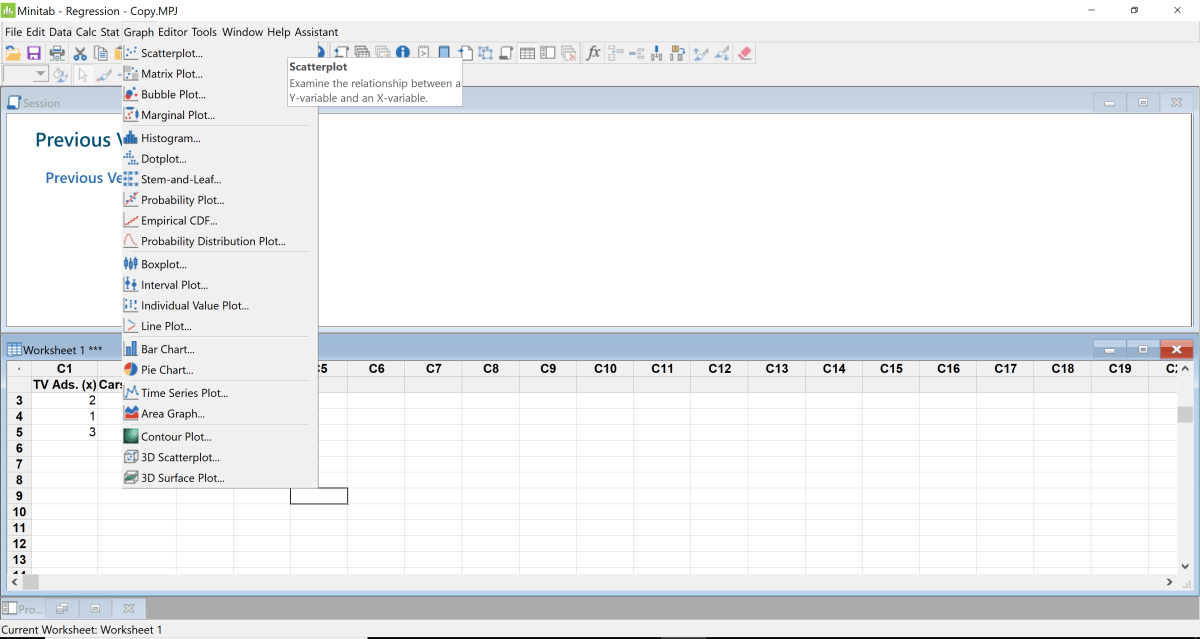Click the tab graph and select Scattered Plot.Created by Joshua Crowder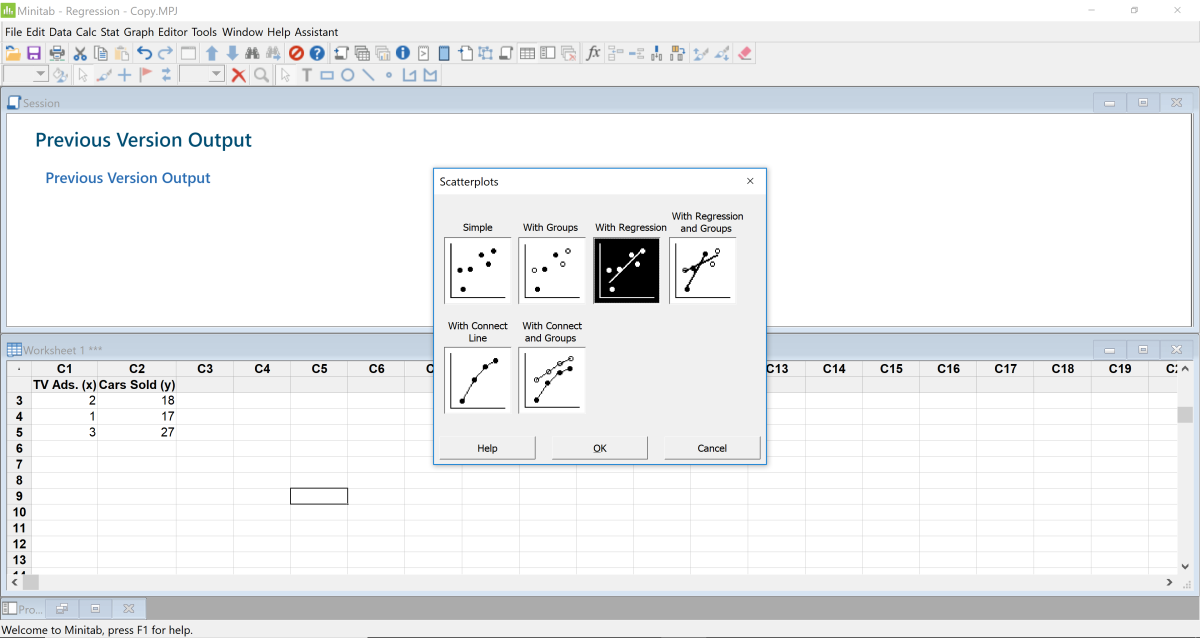Select With Regression.Created by Joshua Crowder
Scroll to Continue

## Set up Fit Regression Model

1. To set up additional regression graphs, first click Data, then click Regression, then Regression, then Fit Regression Model.
2. Now you must place your cursor in the "Responses" section and click on the cars sold header (Y variable) to the left. Click in the "Continuous predictors" section and then click on the TV Ads header (The x variable). Find the storage button and click on it.
3. From the checkboxes, select Fits, Standardized residuals, and Coefficients. Click Okay.
4. There is one more task that will allow us to show multiple residual graphs. Click on Regression Graphs and choose the option "Four in one." Now click Okay. Then click OK again.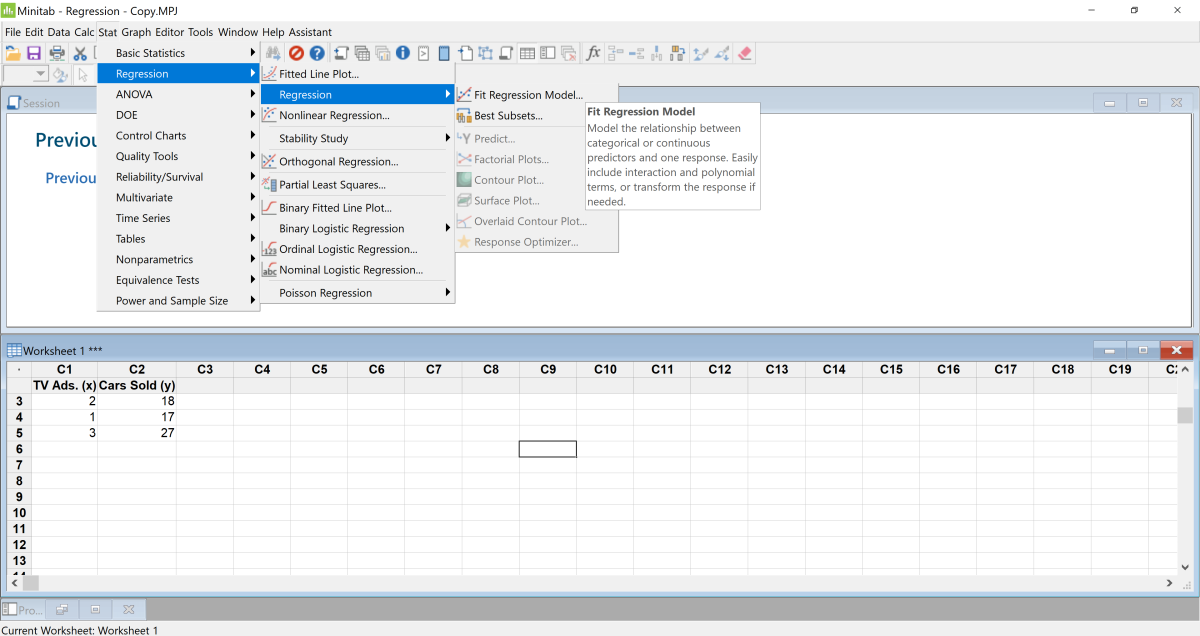Click Data, Regression, Regression, Fit Regression Model.Created by Joshua Crowder

## Scattered Plot, Residual Plots, and Regression Output Data

The scatter plot shows us that the number of cars sold is directly related to the amount of TV advertising. This can be visibly seen without having the regression displayed in the graph. The residual plots graphically display the difference between the observed value of the dependent variable (y) and the predicted value (x). And finally, the output data shows a numerical analysis of the variance.